# GSEB Solutions Class 7 Maths Chapter 15 Visualising Solid Shapes Ex 15.1

Gujarat Board GSEB Textbook Solutions Class 7 Maths Chapter 15 Visualising Solid Shapes Ex 15.1 Textbook Questions and Answers.

## Gujarat Board Textbook Solutions Class 7 Maths Chapter 15 Visualising Solid Shapes Ex 15.1Question 1.
Identify the nets which can be used to make cubes (cut out copies of the nets and try it):
Solution: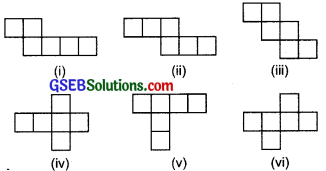(ii), (iii), (iv) and (vi) can be used to make cubes.Question 2.
Dice are cubes with dots on each face. Opposite faces of a die always have a total of seven dots on them.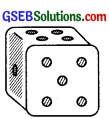Here are two nets to make dice (cubes); the numbers inserted in each square indicate the number of dots in that box.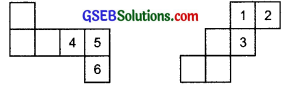Insert suitable numbers in the blanks, remembering that the number on the opposite faces should total to 7.
Solution: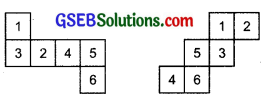Question 3.
Solution: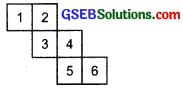No. Because one pair of opposite faces will have 1 and 4 on them whose total is not equal to 7. Another faces are having 3 and 6 on them whose total is also not equal to 7.Question 4.
Here is an incomplete net for making a cube. Complete it in at least two different ways. Remember that a cube has six faces. How many are there in the net here? (Give two separate diagrams. If you like, you may use a squared sheet for easy manipulation.)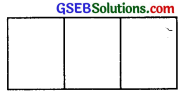Solution: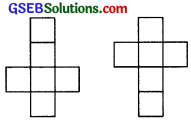Question 5.
Match the nets with approprite solids: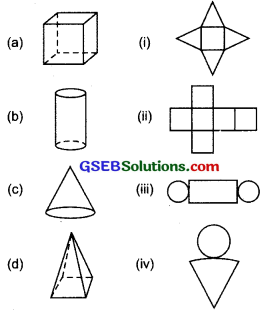Solution:
(a) – (ii)
(b) – (iii)
(c) – (iv)
(d) – (i)